# 2D wave spectrum

HARES can detect and analyse all local wave propagation directions within the HARES solution by means of a 2D wave spectrum. As an example the port of Scheveningen is modelled in HARES. This model consists of 3.4 million elements with a grid size of 1 meter. The computational domain and the applied bathymetry is depicted in the figure at the right side.

Next, a wave condition with a wave height Hm0 of 3.0m, a peak wave period Tp of 10.0s and a wave direction of 330°N was imposed on the circular wave boundary. For this wave condition a JONSWAP spectrum is assumed with gamma=3.3 and a directional spreading of approximately 25° (m=4.0). The wave condition is discretised into 23 frequencies and 17 directions, which amounts to a total of 391 spectral components. This discretisation can be seen in the two figures below.

HARES has a very fast and efficient spectral treatment of bottom friction and wave breaking based on the entire wave spectrum. To do so, all spectral components are computed within one HARES computation and after each iteration step the entire wave spectrum is reconstructed to calculate the amount of bottom friction and wave breaking. The calculated energy dissipation is then equally distributed among all components.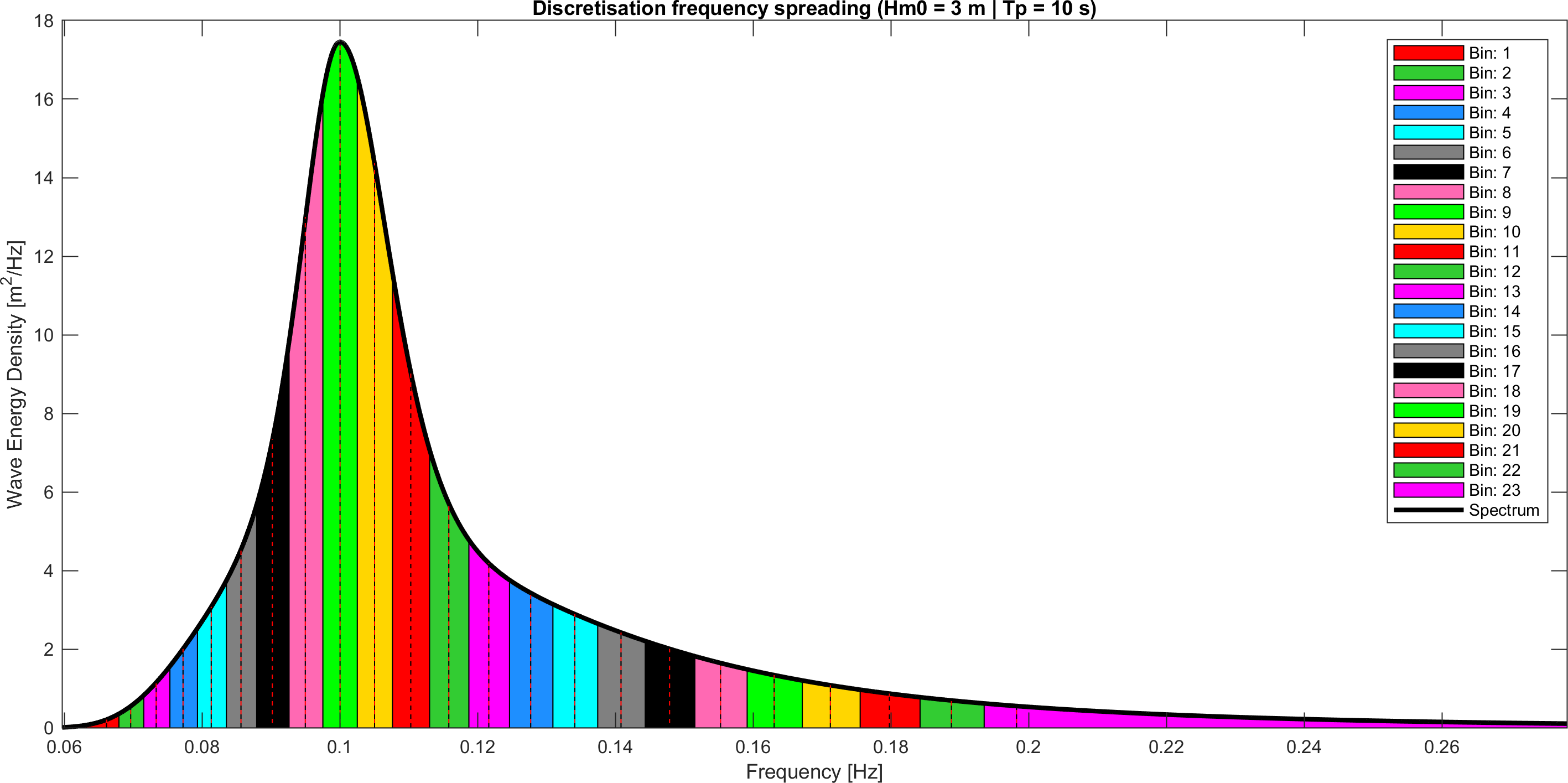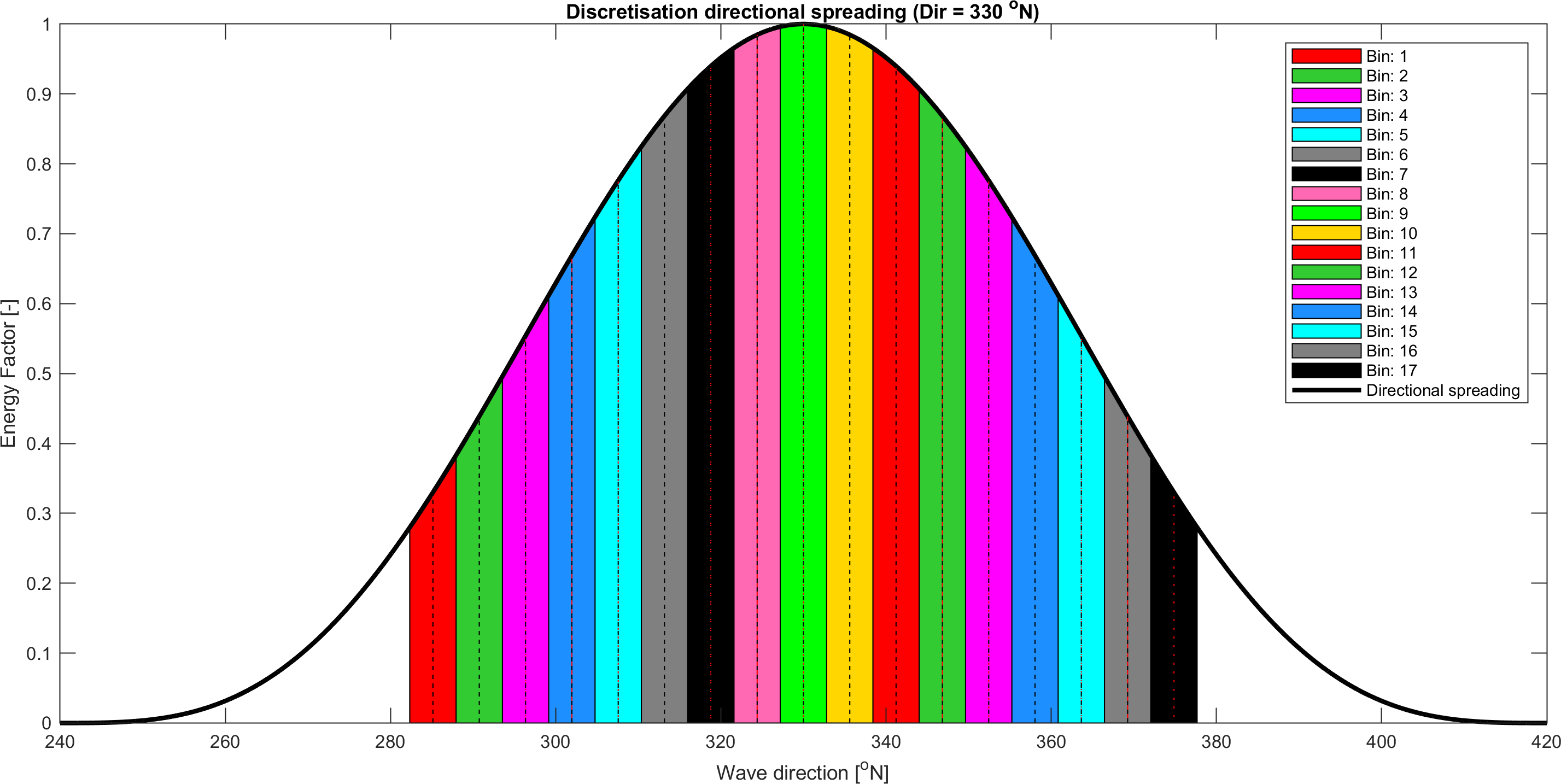The result of the HARES wave penetration run of the imposed wave condition in the port of Scheveningen is shown in the figure at the right hand side. The figure shows the spatial distribution of the wave height Hm0. Four output locations are defined in the model area in which the energy average wave height is determined. As the location is further away from the port entrance, the wave height also decreases.

Below the calculated Hm0, the 2D energy density spectra for all 4 output locations are given. Note that in the spectra, the energy density scale varies per figure.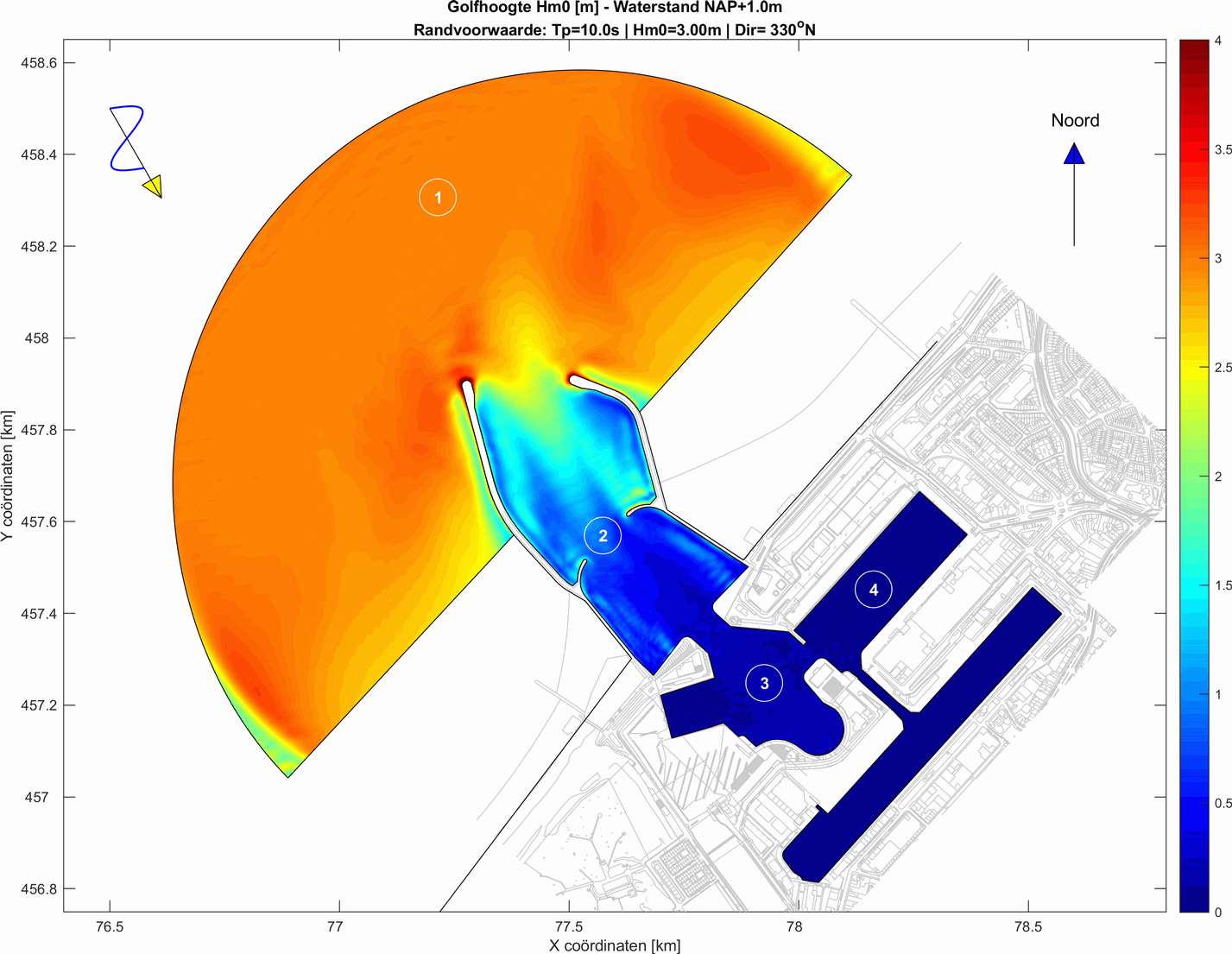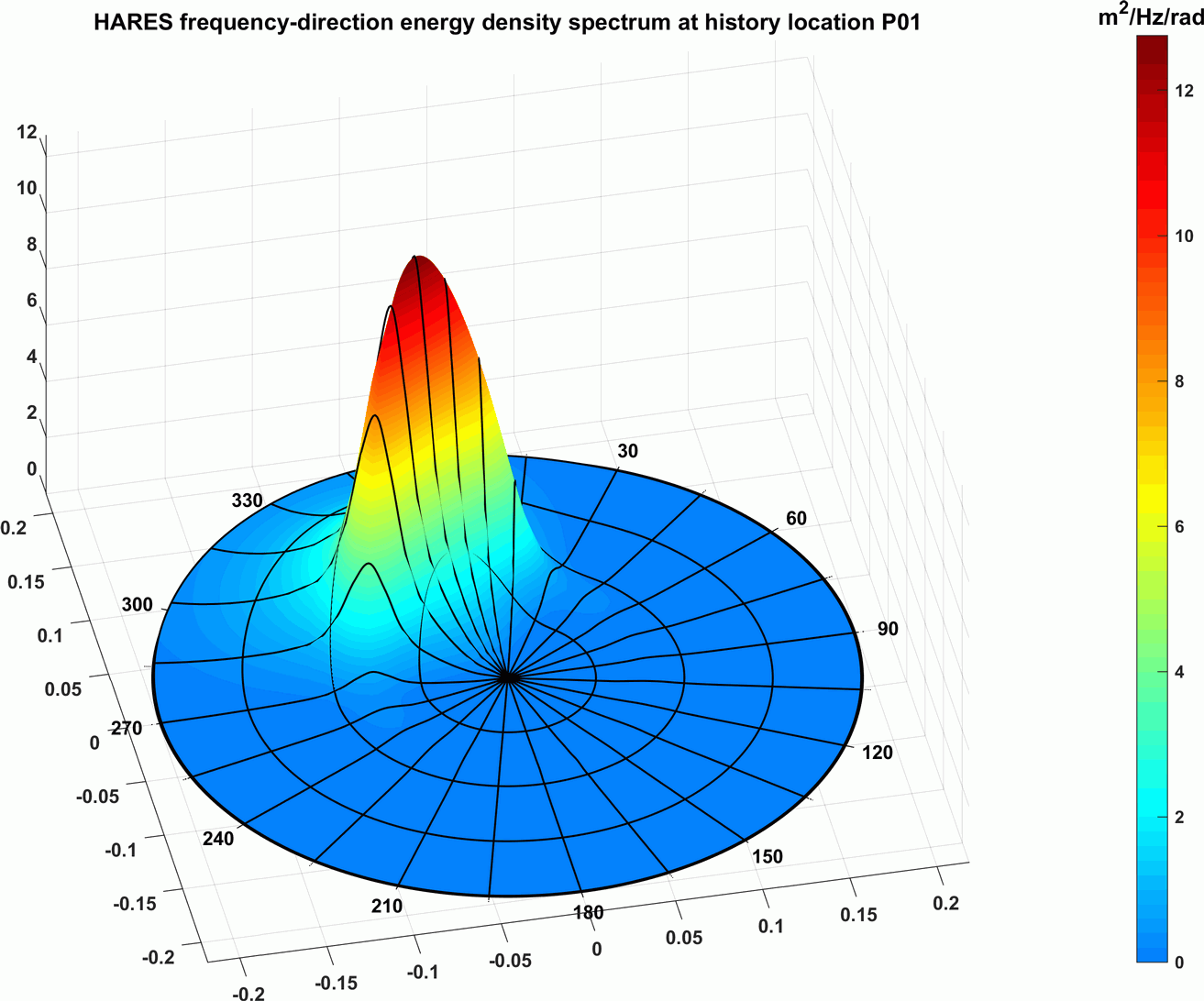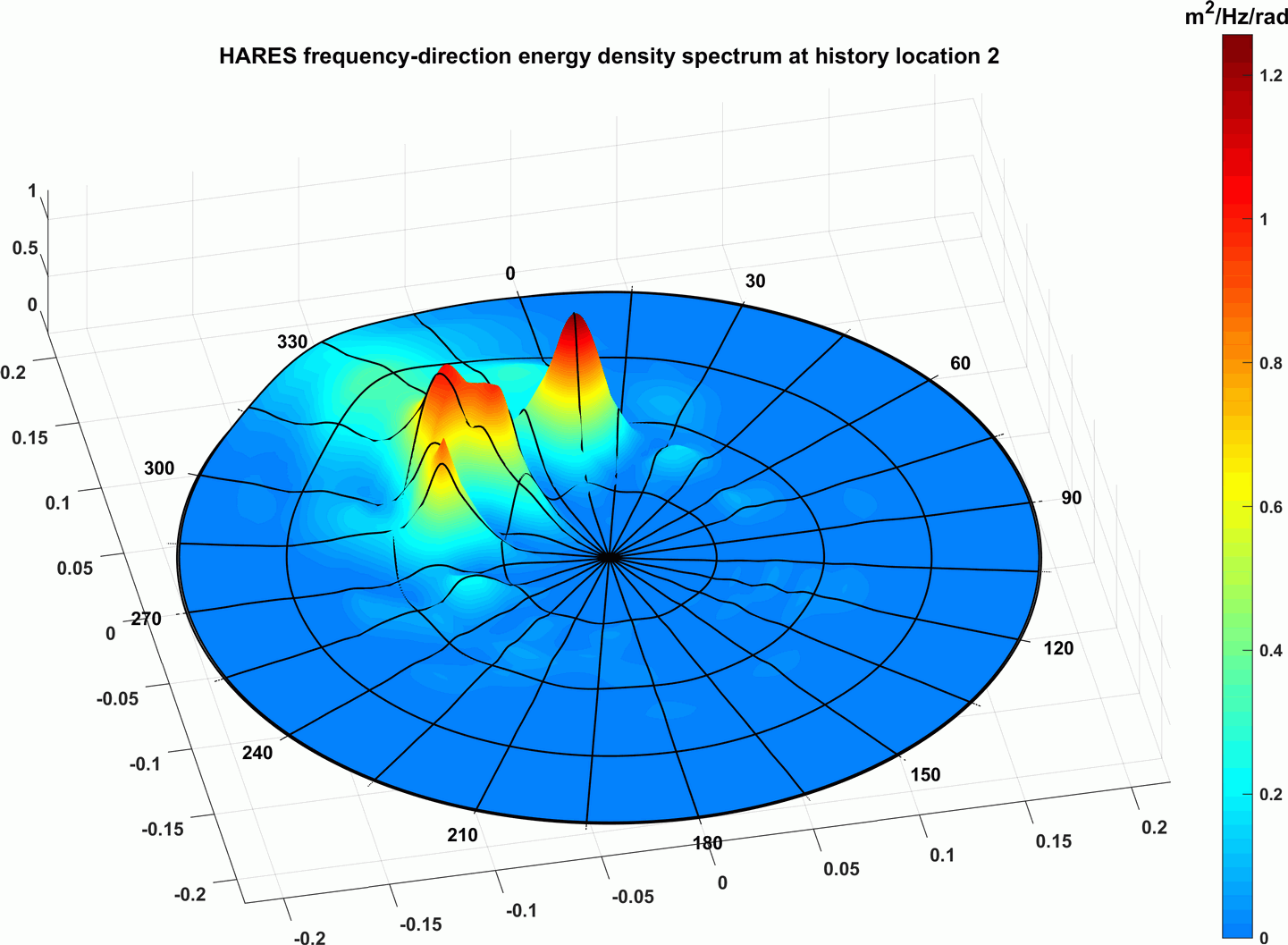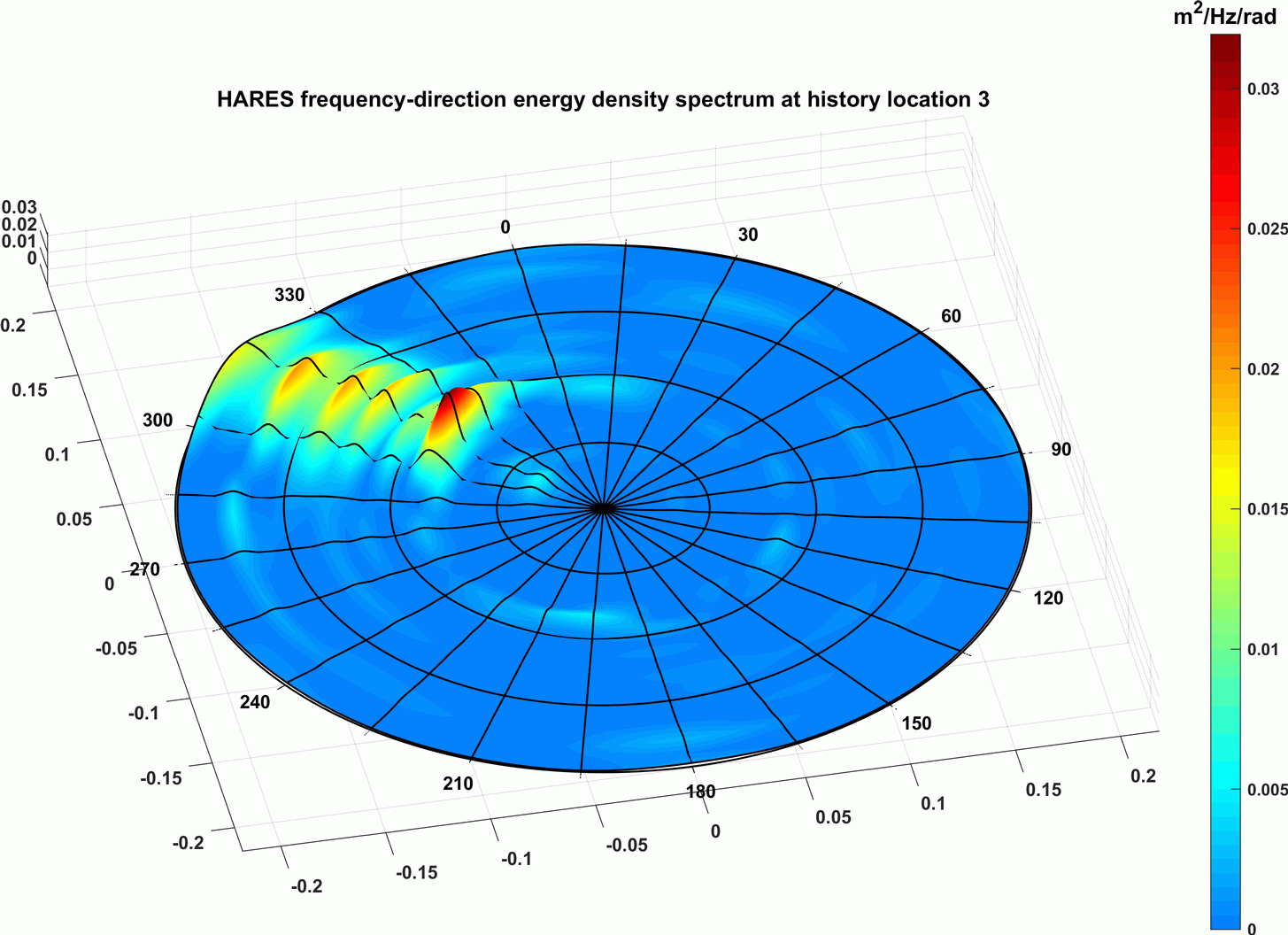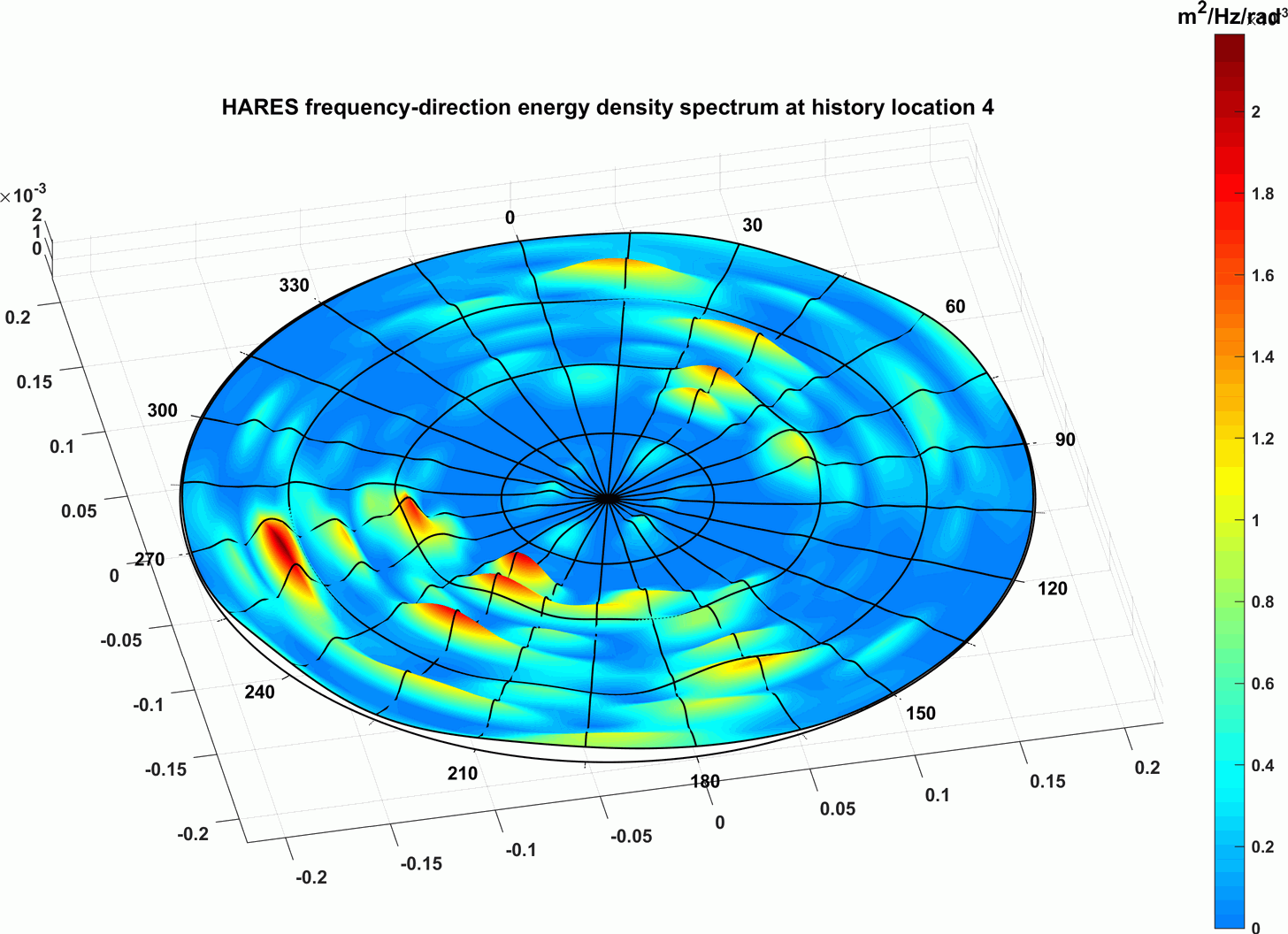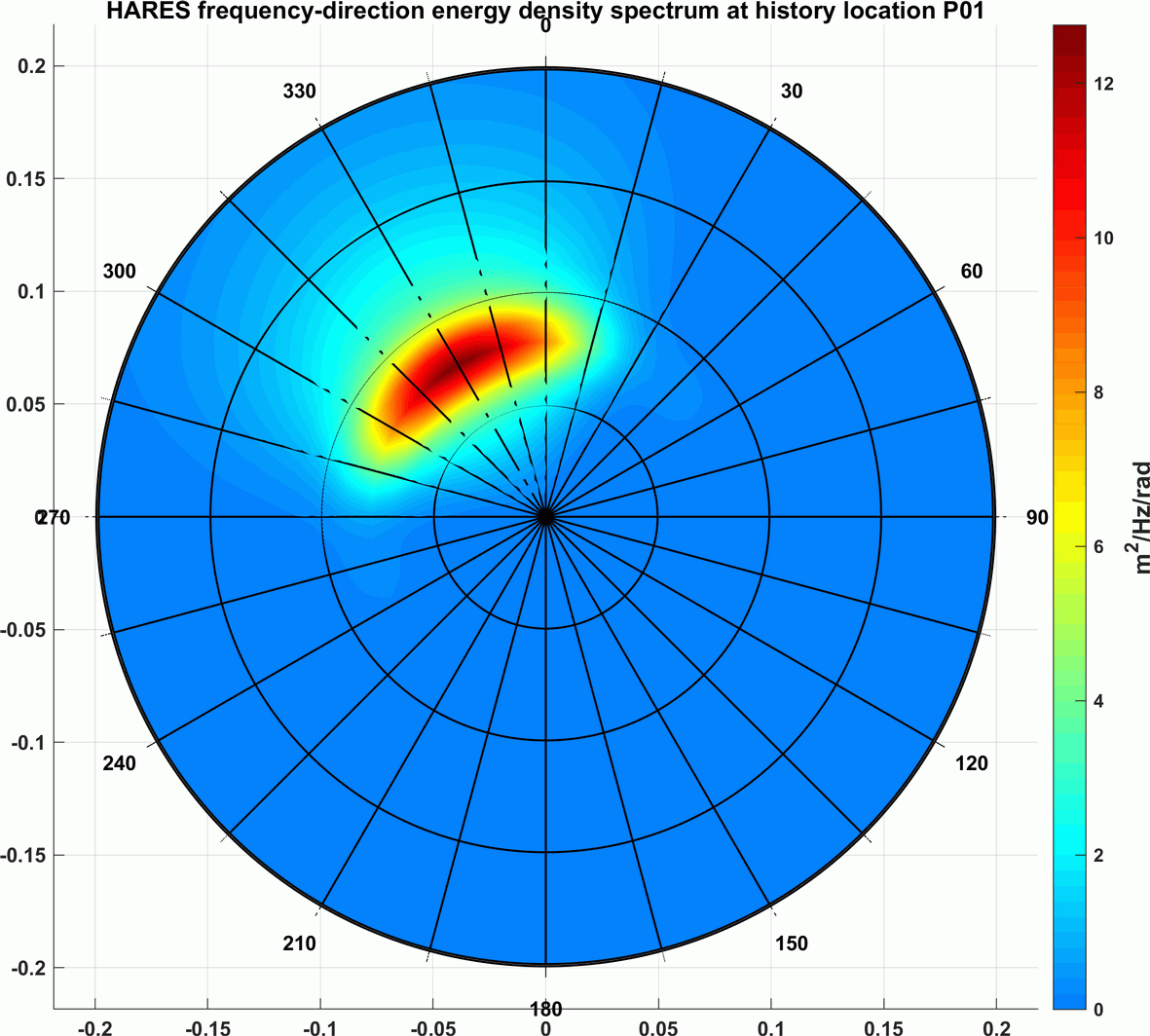Location 1 - Hm0=2.98m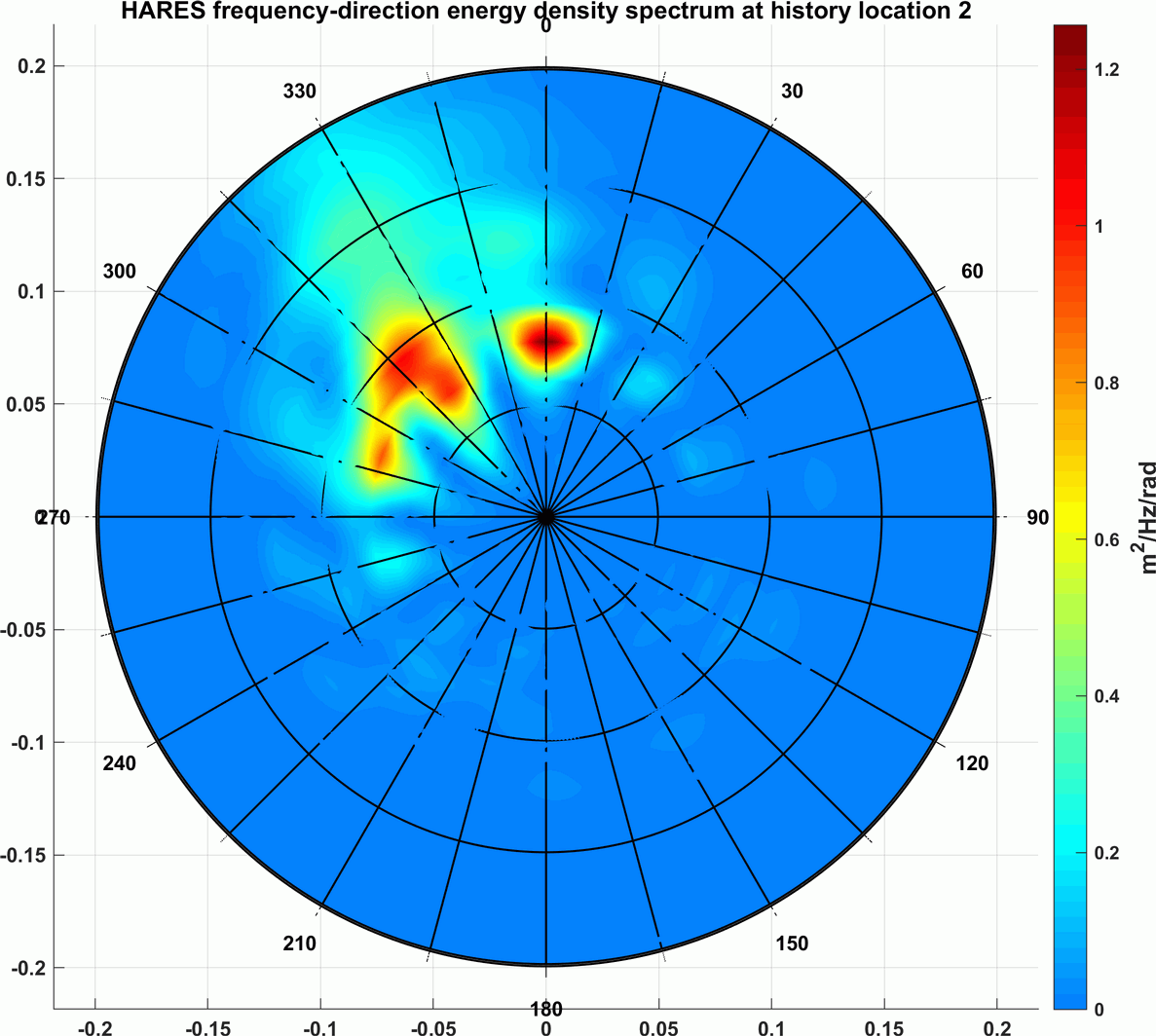Location 2 - Hm0=0.87mLocation 3 - Hm0=0.17m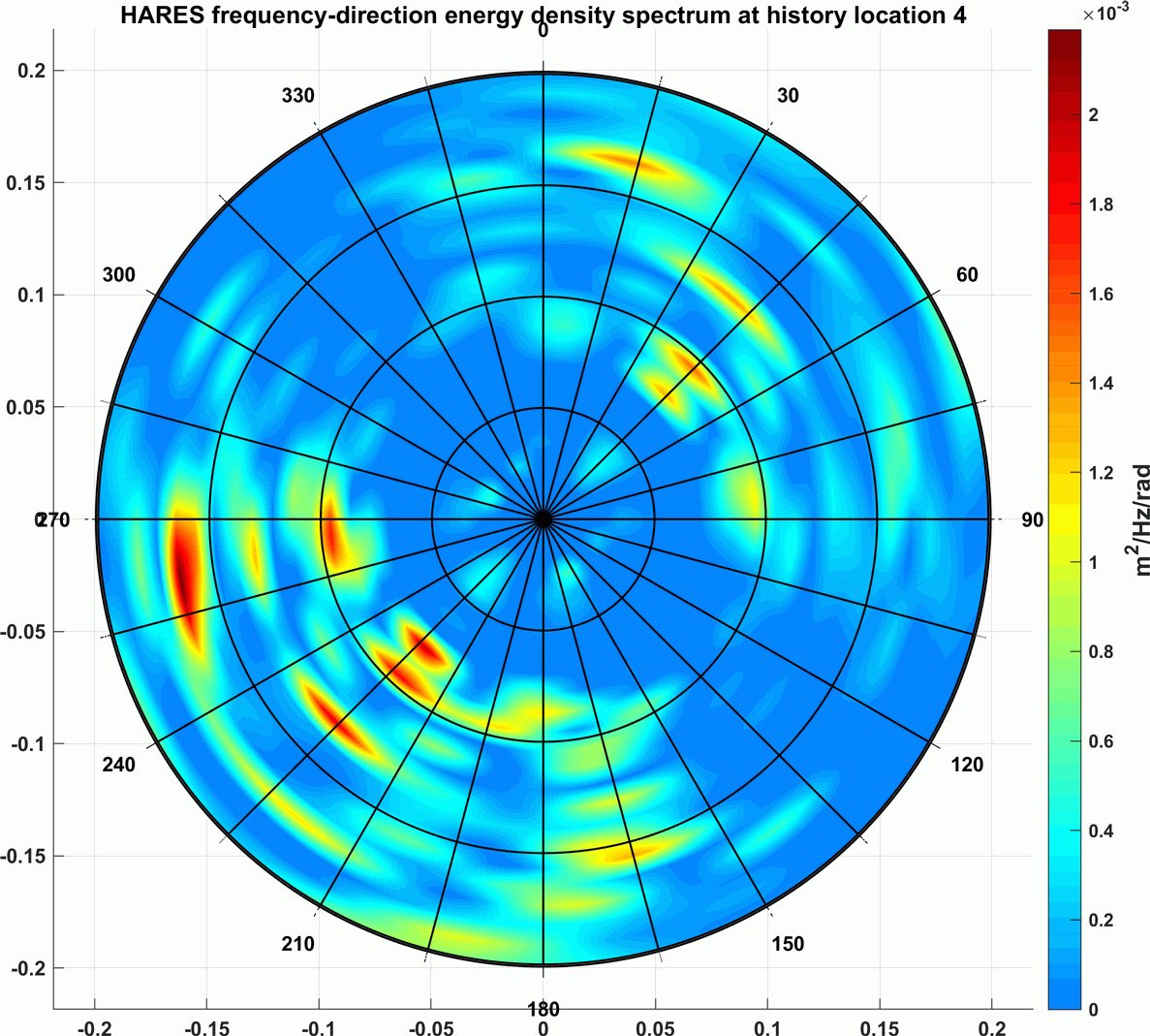Location 4 - Hm0=0.07m